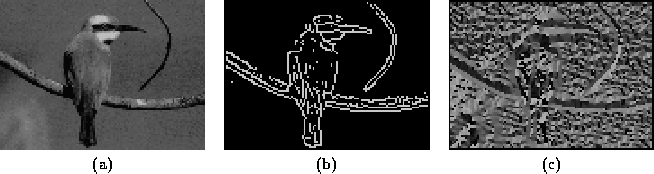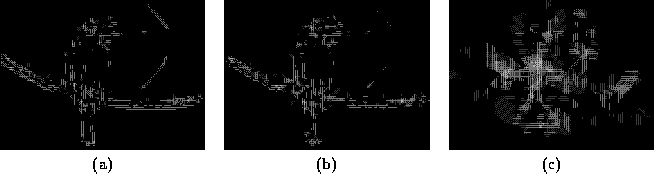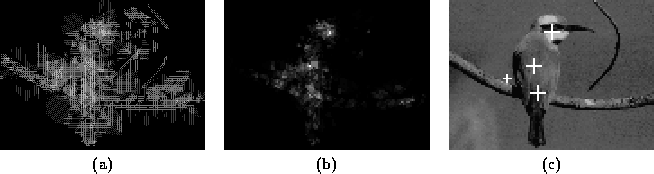Next: Selective Symmetry Detection for Up: Real-Time Symmetry Transform Previous: Dark and Bright Symmetry

## Application

An algorithm very similar to Sela's proposed attentional mechanism is utilized to produce interest maps. These interest maps peak at the intersections of lines of symmetry which occur at the loci of symmetrically enclosed regions or blobs''. Certain modifications to Sela's implementation were made. These include the ability to change the range of the values of r of the annular sampling regions, as well as the ability to select between dark, bright and general symmetry. Furthermore, the symmetry lines are kept as an output of the algorithm since they will be utilized to identify limbs''. Limbs are symmetric structures with a single salient line of symmetry. Since this single axis is not intersected by other lines of symmetry, limbs do not generally trigger a strong response in the interest map. However, Kelly  claims that limbs can have significant perceptual significance despite this. Consequently, the intermediate data are very useful in the extraction of elongated limb-like structures, as proposed by Kelly. Limb extraction will be illustrated in the Chapter 3 as a technique for extracting the mouth from a face.

The following illustrates a typical application of the algorithm to compute the general symmetry transform of an image. Figuredisplays the input to the algorithm. The lines of symmetry are computed over all 8 scales (i.e. at all 8 values of r) using Equation. Figureshows the resulting line segments or points of symmetry which must be linked to form continuous lines of symmetry. The scales used for these symmetry maps are r=1, r=2 and r=8. Figureshows the effect of Equationwhich combines the 8 separate maps by selecting the maximum response from r = 1 to 8. The desired interest map I(p) is also shown in Figure. The points in I(p) undergo Gaussian smoothing and local maximum detection  to generate a set of discrete interest points at the centers of clusters of response found in I(p). The resulting interest points are finally displayed superimposed upon the input image.Next: Selective Symmetry Detection for Up: Real-Time Symmetry Transform Previous: Dark and Bright Symmetry
Tony Jebara
2000-06-23